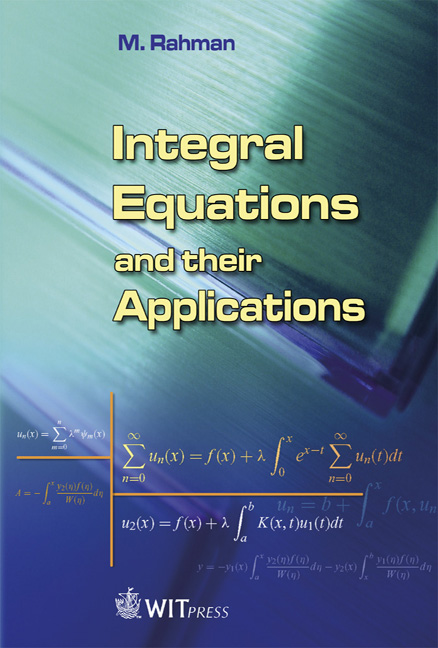WIT Press# Integral Equations and their Applications

Authors: M. Rahman, Dalhousie University, Canada

Price

\$290.00 (free shipping)

ISBN

978-1-84564-101-6

eISBN

978-1-84564-289-1

Pages

384

Published

2007

Format

Hardback

For many years, the subject of functional equations has held a prominent place in the attention of mathematicians. In more recent years this attention has been directed to a particular kind of functional equation, an integral equation, wherein the unknown function occurs under the integral sign. The study of this kind of equation is sometimes referred to as the inversion of a definite integral.

While scientists and engineers can already choose from a number of books on integral equations, this new book encompasses recent developments including some preliminary backgrounds of formulations of integral equations governing the physical situation of the problems. It also contains elegant analytical and numerical methods, and an important topic of the variational principles. Primarily intended for senior undergraduate students and first year postgraduate students of engineering and science courses, students of mathematical and physical sciences will also find many sections of direct relevance.

The book contains eight chapters, pedagogically organized. This book is specially designed for those who wish to understand integral equations without having extensive mathematical background. Some knowledge of integral calculus, ordinary differential equations, partial differential equations, Laplace transforms, Fourier transforms, Hilbert transforms, analytic functions of complex variables and contour integrations are expected on the part of the reader.